## How can I make a map to solve equation knowing all...

I make a function
myf := (a, b, c) -> [a*x^2 + b*x + c = 0, solve(a*x^2 + b*x + c = 0, {x})];

I tried

myf(1, 2, -3);

and get

[x^2 + 2*x - 3 = 0, {x = 1}, {x = -3}]

With list mylist := [[1, 2, -3], [3, 2, -1]];

How can I map myf  onto mylist?

I tried

myf := (a, b, c) -> [a*x^2 + b*x + c = 0, solve(a*x^2 + b*x + c = 0, {x})];
mylist := [[1, 2, -3], [3, 2, -1]];
map(myf, mylist);

I can not get the result.

## max, max[index] for tables?...

Apparently max and max[index] don't work with tables.

I'd like to put that on a wish list for future development.

Right now the workaround for the first one would be max(entries(atable)) I think.

Haven't solved max[index] yet.

## Is that a graphics card artefact...

I was wondering whether the rendering of bars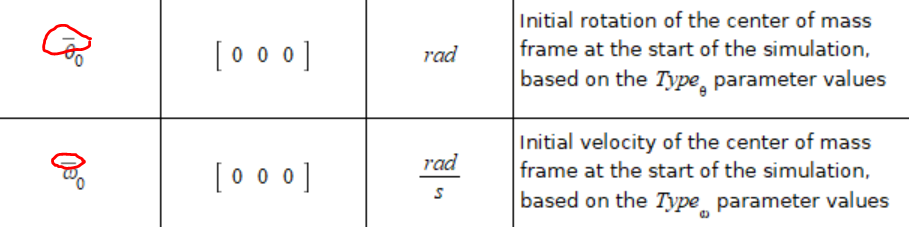looks also dot like on other computers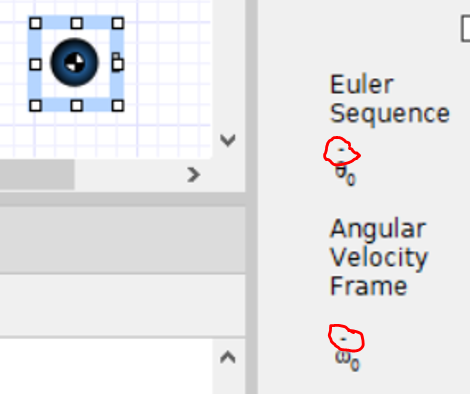Looks like Newtonian notation

## Question on Fourier Transforms...

When the Fourier transform to the heat equation for example it is useful to represent the Fourier transform of a derivative as the derivative of the transform.  Thus

FT{d(U(x, t)/dt}  = d(FT{U(t))} / dt,

Where FT => Fourier transform wrt the variable x.

How do I get Maple to obtain the above.

Joe Salacuse

## Simplifying expression with sqrt of complex expres...can be simplified :l *h*t/m

How can I ask Maple to do this.

## trick to show this expression is zero?...

Given

expr:=  (x^(-(44 + 12*sqrt(69))^(1/3)/6 + 10/(3*(44 + 12*sqrt(69))^(1/3)) + 2/3)) - (x^(sqrt(69)*2^(1/3)*((11 + 3*sqrt(69))^2)^(1/3)/100 - (11*(44 + 12*sqrt(69))^(2/3))/600 - (44 + 12*sqrt(69))^(1/3)/6 + 2/3))

could someone come with a trick to show this is zero using simplification and other methods? I know I can use is and coulditbe but I do not trust these too much due to false positives I've seen from them in some places.

Here is my attempts

restart;

expr:=  (x^(-(44 + 12*sqrt(69))^(1/3)/6 + 10/(3*(44 + 12*sqrt(69))^(1/3)) + 2/3)) - (x^(sqrt(69)*2^(1/3)*((11 + 3*sqrt(69))^2)^(1/3)/100 - (11*(44 + 12*sqrt(69))^(2/3))/600 - (44 + 12*sqrt(69))^(1/3)/6 + 2/3));
simplify(expr);
simplify(expr,size);
simplify(expr,symbolic);
simplify(normal(expr));
simplify(normal(expr),symbolic);
simplify(expr) assuming real;
simplify(expr) assuming positive;
is(expr=0);
coulditbe(expr=0);
evalb(expr=0);


Gives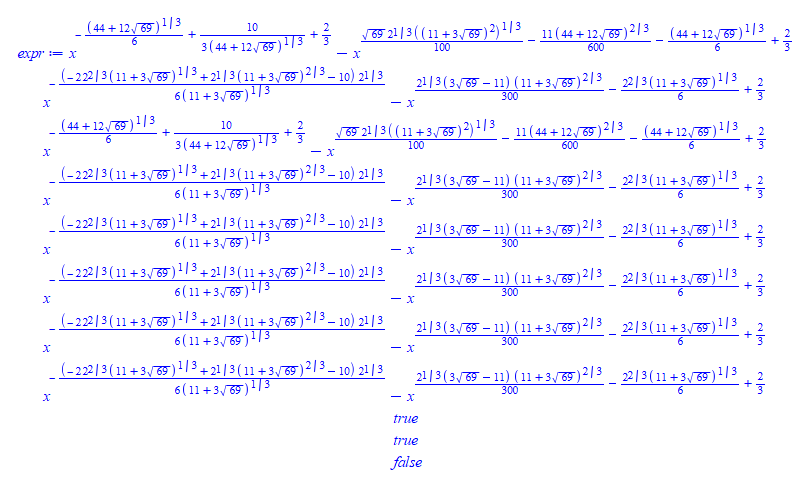This is for reference, the Mathematica attempt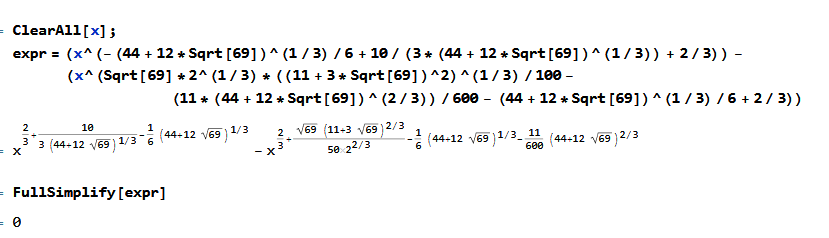## Latex problem with spacing before dx. Is there an ...

long time ago I asked about automatic spacing to improve latex for sqrt. A nice solution was provided in https://www.mapleprimes.com/questions/231062-Adding-Space-After-Sqrt-To-Improve-The-Latex

The above is activatived using spaceaftersqrt = true option for latex:-Settings

There is a similar issue for inert integration where a space is typically added before the final dx This is done similar to the above fix using \, See for example this Latex web site giving many examples.

But there is no option I could see to tell Maple to do this automatically for integration.

So all my inert integrals now look not too good as the dx is too close to the integrand. Here are 2 examples with the settings I am using

restart;

latex:-Settings(useimaginaryunit=i,
usecolor = false,
powersoftrigonometricfunctions= mixed, ## computernotation,
leavespaceafterfunctionname = true,
cacheresults = false,
spaceaftersqrt = true,
usetypesettingcurrentsettings=true,
linelength=10000
);

sol:=sqrt(4*y^3-a*y-b)*a;
Intat( subs(y=a,1/sol),a=y(x));
latex(%);

Int(sol,y);
latex(%);


I copied the latex and compiled it, and this is the result

\documentclass[12pt]{book}
\usepackage{amsmath}
\begin{document}

$\int_{}^{y \left(x \right)}\frac{1}{\sqrt{4 a^{3}-a^{2}-b}\, a}d a$

$\int \sqrt{4 y^{3}-a y -b}\, a d y$

\end{document}

Which gives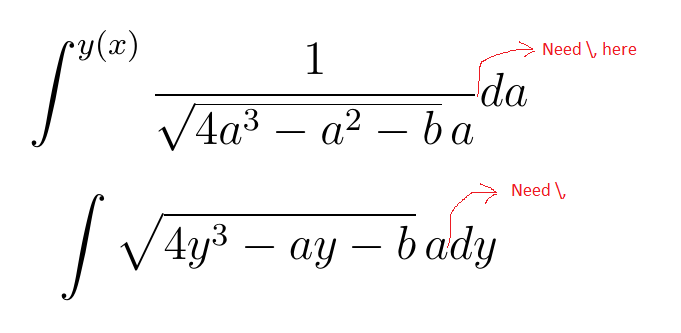Compare the output when adding \, by hand to the latex

\documentclass[12pt]{book}
\usepackage{amsmath}
\begin{document}

$\int_{}^{y \left(x \right)}\frac{1}{\sqrt{4 a^{3}-a^{2}-b}\, a}\, d a$

$\int \sqrt{4 y^{3}-a y -b}\, a \, d y$

\end{document}

Which now gives much better result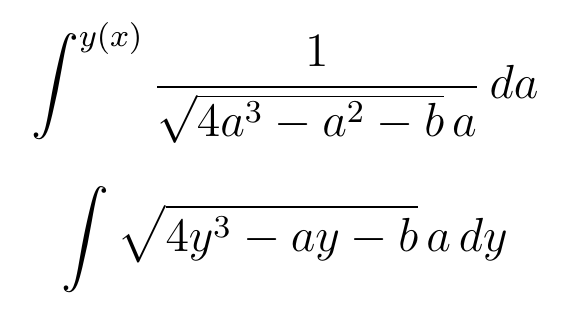Actually, what would be nice if the "d" in "dx" was mathrm which becomes

\documentclass[12pt]{book}
\usepackage{amsmath}
\begin{document}

$\int_{}^{y \left(x \right)}\frac{1}{\sqrt{4 a^{3}-a^{2}-b}\, a}\, \mathrm{d} a$

$\int \sqrt{4 y^{3}-a y -b}\, a \, \mathrm{d} y$

\end{document}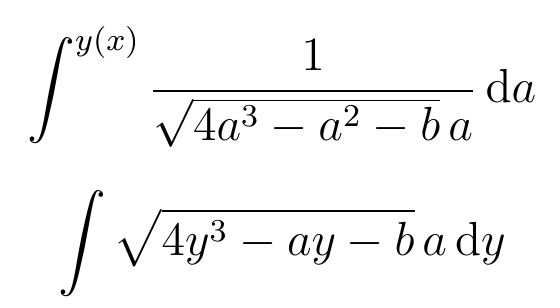But may be I am asking for too much here. But having an option to add \, only for inert integration will be good to have.

Does there exist an option to do this that may be I overlooked?

Maple 2023.2

## Why does fsolve only provide one solution?...

I have the function: 2*sin(x)+1/2x-1

I want the x-intercepts, but Maple only provides one answer with fsolve.## Where is wrong in this code?...

I am trying to find the integer numbers a, b, c, d, t so that the equation sqrt(a x^2 + b x + c) = d x + t have two integer solutions. My code

First I solve

solve(a*x^2 + b*x + c = (d*x + t)^2, x);

I get

(2*d*t - b + sqrt(4*a*t^2 - 4*b*d*t + 4*c*d^2 - 4*a*c + b^2))/(2*(-d^2 + a)), -(-2*d*t + sqrt(4*a*t^2 - 4*b*d*t + 4*c*d^2 - 4*a*c + b^2) + b)/(2*(-d^2 + a))

And then, I solve
restart;
n := 0;
for a to 10 do
for b to 10 do
for c to 10 do
for d to 10 do
for t to 10 do
mydelta := 4*a*t^2 - 4*b*d*t + 4*c*d^2 - 4*a*c + b^2;
if 0 < mydelta and type(mydelta, integer) then
x1 := (2*d*t - b + sqrt(4*a*t^2 - 4*b*d*t + 4*c*d^2 - 4*a*c + b^2))/(2*(-d^2 + a));
x2 := -(-2*d*t + sqrt(4*a*t^2 - 4*b*d*t + 4*c*d^2 - 4*a*c + b^2) + b)/(2*(-d^2 + a));
if 0 < d*x1 + t and 0 < d*x2 + t and type(x1, integer) and type(x2, integer) and nops({a, b, c, d, t}) = 5 and c <> t^2 and -d^2 + a <> 0 and nops({x1, x2}) = 2 then n := n + 1; L[n] := [a, b, c, d, t]; end if; end if; end do; end do; end do; end do;
end do;
L := convert(L, list);
nops(L);

I don't get any solutions. But, when I  solve(sqrt(2*x^2 + 3*x + 5) = x + 9, x); I get two solutions 19, -4.
Where is wrong in my code?

## equality of vectors (too subtle for me)...

S := [1, 2];
S := [1, 2]

T := [1, 2];
T := [1, 2]

is(S = T);
true

Sv := Vector([1, 2]);

Tv := Vector([1, 2]);

is(Sv = Tv);
false

is(convert(Sv, list) = convert(Tv, list));
true

## first order pde...

how to solve tis type of pdefirst_order_pde.mw

## why has works different on seemingly same input?...

I obtained a Plot p, then trying to find if it has Float(undefined) in it. I am basically trying to find if DEplot returns an empty plot or not. I found that it the plot structure has HFlat(undefined) then this seems to imply an empty plot. So I said, then in the code I can check for HFlat(undefined) in the plot and it is there, then I know the plot is empty.

But this check is not working.

I used has(....,Float(undefined)) and this returns false. But if I copy and paste part of the plot structure and try the check on the copy, it gives true.

I do not understand what is the difference. First here is screen shot, and below if complete code to reproduce.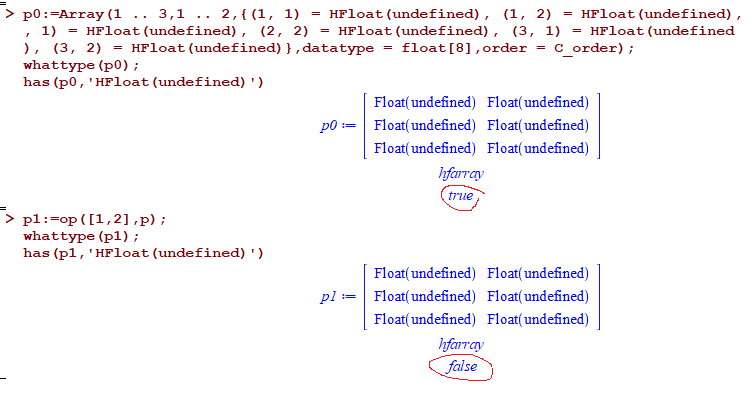restart;
ode2:=2*y(t)+t*diff(y(t),t) = t^2-t+1;

p:=DEtools:-DEplot(ode2,y(t),t=0..3.5,y=0..3,
linecolor = red,
arrowsize ='magnitude',
numpoints = 200 ,
axes = boxed,color = ('magnitude[legacy]')):

p0:=Array(1 .. 3,1 .. 2,{(1, 1) = HFloat(undefined), (1, 2) = HFloat(undefined), (2
, 1) = HFloat(undefined), (2, 2) = HFloat(undefined), (3, 1) = HFloat(undefined
), (3, 2) = HFloat(undefined)},datatype = float,order = C_order);
whattype(p0);
has(p0,HFloat(undefined))

p1:=op([1,2],p);
whattype(p1);
has(p1,HFloat(undefined))



Worksheet attached also.

Maple 2023.2 on windows 10> restart;> ode2:=2*y(t)+t*diff(y(t),t) = t^2-t+1; p:=DEtools:-DEplot(ode2,y(t),t=0..3.5,y=0..3,                         linecolor = red,                                                 arrowsize ='magnitude',                         numpoints = 200 ,                         axes = boxed,color = ('magnitude[legacy]')):> p0:=Array(1 .. 3,1 .. 2,{(1, 1) = HFloat(undefined), (1, 2) = HFloat(undefined), (2 , 1) = HFloat(undefined), (2, 2) = HFloat(undefined), (3, 1) = HFloat(undefined ), (3, 2) = HFloat(undefined)},datatype = float,order = C_order); whattype(p0); has(p0,HFloat(undefined))> p1:=op([1,2],p); whattype(p1); has(p1,HFloat(undefined))>

question is: Why the above give different result? And what is the correct way to check if the output plot from DEplot is empty or not (in code)?